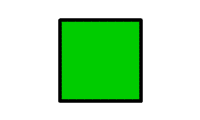# The Shape-Shifting Triangle- Part 2

Geometry Level 3Say the area of an isosceles triangle with two sides, each equal to $3$ units, is $A$. Find the maximum value of $A^{2}$. Write the answer to two decimal places.

Try Part 1

×